## Week 2 Coding Lecture 3: Functions

Throughout this class, and throughout applied mathematics in general, we frequently need to work with mathematical functions like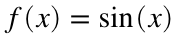or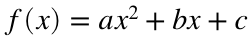. As an example, in the activity this week you will write code that calculates the roots of these functions (i.e., values of x such that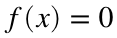). As you might imagine, the formula for the appropriate function will appear in numerous places in your code. Some functions (such as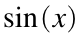) are pre-defined in MATLAB, but many other functions (such as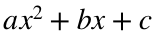) are not. This can be problematic, because it means we have to write out the formula for those functions in many different places. Moreover, if we decide to change the formula then we will have to change many different lines of code, which is one of the most reliable ways to introduce typos and bugs into your code.
We already know a very common solution to this problem in mathematical notation: If we want to work with a complicated formula, we just give it a name. This is what we mean when we writeor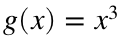. The name f or g is a replacement for the full formula.
Ideally, we would like to be able to apply the same idea to code. In particular, it would be nice to say something like f = sin(x) or f(x) = sin(x), and then be able to use the variable f in place of the formula. Unfortunately, this exact syntax would not do what we want. The line
f = sin(x);
would either cause an error (because x is not defined) or set f equal to a number (because MATLAB will plug the value of x into sin). Similarly, the line
f(x) = sin(x);
will not work as intended. In this case, you might see a wide variety of errors, depending on whether f or x are already defined and what their exact values are. Likewise, the line
f = sin;
will produce an error. Our idea, however, is sound; we just need to use somewhat more awkward syntax.

### Review

Before we talk about h ow to write a function, let's briefly review what they are and how to use them. You have already seen quite a few MATLAB functions. For example, sin and cos are functions, as are zeros and why. MATLAB functions are just prewritten chunks of code that you can run by using their name. You can tell MATLAB to run a function by writing the function name followed by parentheses and a list of some number of input values called "arguments". This process is known as "calling" the function. For example, the function why does not need any arguments, so you can call it by typing the name and a set of parentheses:
why()
To please a very terrified and smart and tall engineer.
The function sin takes one argument (an angle in radians), so you can call it by typing the name and then a number in parentheses:
sin(4)
ans = -0.7568
The function zeros can take any number of arguments, so you can call it by typing the name and some number of values in parentheses, each separated by a comma. For instance:
zeros(3)
ans = 3×3
0 0 0 0 0 0 0 0 0
zeros(2, 5)
ans = 2×5
0 0 0 0 0 0 0 0 0 0
Functions can also have an output (called a "return value"). If a function has a return value, you can assign that value to a variable when you call the function. For instance, sin has a return value, which is the sine of the argument in radians. As we have already seen, you can assign the return value of sin to a variable by writing
x = sin(4)
x = -0.7568
Similarly, the zeros function has a return value. In this case, the return value is a matrix of all zeros. You can assign this matrix to a variable like so:
A = zeros(2, 3)
A = 2×3
0 0 0 0 0 0
Some functions don't have return values, so you can't assign them to a variable. For instance, you cannot write
x = why()
or
z = disp(10)
because why and disp do not have any return values.
Some functions can also have more than one return value, but we have not encountered any examples yet.

To define your own function, you need to specify the function name, any arguments and return values, as well as the code that MATLAB will run when the function is called. There are three different ways to write a function in MATLAB (well, there are a few more related versions, but we will only use these three): Anonymous functions, local functions and function files. Each of these has a slightly different syntax and can be used in slightly different ways.

### Anonymous functions

The simplest type of function is called an "anonymous function". You can define it with the following syntax:
function_name = @(list_of_argument_names)(one_line_of_code)
For example, we can define the function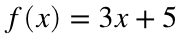with the code
f = @(x)(3 * x + 5)
f = function_handle with value:
@(x)(3*x+5)
You can now call this function by writing its name followed by a value of x in parentheses. For example:
f(4)
ans = 17
y = f(3)
y = 14
z = f(3) - f(2) + 3
z = 6
• f is a variable in the workspace. Its type is function_handle, not double. If you double click on it, you will be able to see the formula you entered. Since this variable is in the workspace, you can call the function from the command window or from other scripts. It is not limited to the script where you defined it.
• x is not a variable in the workspace. (Actually, this may or may not be true for you because you might have already defined x somewhere else, but x does not have to be a variable in the workspace. You can prove this to yourself by first using clear all and then redefining f.) This is very important - arguments like x can be used in the code for the function even though they are not already defined.
• When you call a function, the argument you give in parentheses is used as the value of x. For instance, if you write f(4), then MATLAB will use 4 as the value of x.
• The return value is whatever the one line of code (in the second set of parentheses) evaluates to. If you write f(4), then the return value is 3*4 + 5, which is 17. In particular, there is no way to name this value or to make an anonymous function with no return values or more than one return value.
It's also worth noting that the function_name is optional (which is why these are called "anonymous"). It will occasionally be useful to create a function without actually naming it, such as
@(x)(x^2 + 3)
ans = function_handle with value:
@(x)(x^2+3)
The behavior of the argument x might seem particularly strange. As we already saw, defining this function does not define a variable named x. Moreover, even after you call the function, you will not see a variable named x in the workspace. Look at the workspace after this code:
clear all
f = @(x)(3 * x + 5);
y = f(10);
Both f (the function) and y (the return value after you call the function) are defined and in the workspace, but x is not. What's more, if you try this code:
clear all
x = 2;
f = @(x)(3*x + 5);
y = f(10);
x
x = 2
you can see that defining/calling f does not affect any other variables named x. This is because the arguments of the function have something called local scope. The variable x can only be seen by MATLAB while it is running f, and x will not interfere with any variables that aren't in the function body. In essence, MATLAB gives f its own private workspace whenever you call it. For instance, when MATLAB executes the line "y = f(10)" above, it will make a private workspace for f with a variable named x that has the value 10. This private workspace is completely separate from the normal workspace.
The workspace you can see in your main MATLAB window represents something called the global scope. As we have already seen, code from any script or the command window can access and modify variables in the global scope.
The above description of the local scope is actually not quite complete. MATLAB actually gives the function a private workspace which includes a copy of the global scope from the time when the function was created. This means that the function can access (but not modify) variables from the global scope that were available when you defined the function. This fact that this workspace is copied upon function creation can lead to some strange behavior. For example,
clear all
a = 2;
f = @(x)(a * x);
f(5)
ans = 10
When you call the function, MATLAB is able to look up the value of a even though it is not an argument, because it was in the global scope when we created f. However, changes to a after defining the function will not affect the local scope of f. For example,
a = 10;
f(5)
ans = 10
Even though we changed a to 10, the function call is still using the old value of a. As a general rule, this last example is not the sort of behavior you want to rely on. Using this feature intentionally will often result in code that is very difficult to understand. However, there will be several examples later in this class where we can't avoid this issue.
It's also worth noting that the name of the argument does not matter, as long as you are consistent. That is, if you use the name x in the argument list then you also need to use the name x when you refer to that argument in the function body. For example, the following are exactly equivalent:
f = @(x)(3 * x + 5);
f = @(z)(3 * z + 5);
f = @(this_is_a_silly_variable_name123)(3 * this_is_a_silly_variable_name123 + 5);
However,
f = @(x)(3 * y + 5);
will not do what you want. (If y is not yet defined, then this will cause an error. If y is already defined, then every call of f will use the same value of y and just ignore the argument x.)
No matter which (valid) definition you use, you can call the function in the same way:
f(3)
ans = 14
It's also worth noting that you aren't restricted to just using hard coded numbers as arguments when you call a function. Anything that evaluates to a number is fine. For example:
x = 4; y = pi;
f(x)
ans = 17
f(y)
ans = 14.4248
f(x - 2 * y)
ans = -1.8496
Again, notice that there is no confusion with variable names, even though we used x in the definition of f and also used it when calling the function. The x you defined outside the function is completely separate from the argument in your function definition.
You can use this syntax to define functions with any number of arguments. For instance,
f = @(x, y)(x^2 + y);
f(2, 3)
ans = 7
f(3, 2)
ans = 11
g = @(a, b, c, x, y, z)(a + 2*b - c + x^2 - y^2 + 3*z^3);
g(1, 2, 3, 4, 5, 6)
ans = 641
h = @()(2*pi);
h()
ans = 6.2832
Notice that the order of the arguments matters. In the above example, the first argument of f is used as x and the second argument is used as y. That's why f(2, 3) and f(3, 2) are not the same.

### Local functions

Anonymous functions are useful, but quite limited. The biggest problem is that they are limited to one line of code. More specifically, they are limited to a single expression. This distinction doesn't really matter to us, but it's worth noting that an assignment like x = 3 is not an expression, so you cannot use it in an anonymous function.) In particular, you cannot include an if statement or a loop inside an anonymous function, because thos require more than one line of code. If you want to use a more complicated function in one of your scripts, you can write a local function.
Local functions have the following syntax:
function return_value_name = function_name(list_of_argument_names)
% As much code as you want
end
It is very important to remember that all local functions have to be defined at the bottom of your script. If you put code like this in the middle of a script, MATLAB will refuse to run it. No other code is allowed after a local function except for more local functions. (You can have as many local functions as you want at the bottom of the script, though.) In these notes, I will write examples of functions in the middle of the script, but the actual function definitions will always be at the bottom in their own section labeled "Functions". This is because MATLAB will otherwise refuse to run any of the code in this .mlx file.
As an example, we can make a local function for the formula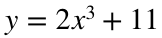with the code
function y = my_first_function(x)
y = 2*x^3 + 11;
end
(Remember, the code right here is marked as an example and so MATLAB ignores it. The actual code is at the bottom of these notes.)
You can now call this function from anywhere in the script using
my_first_function(3)
ans = 65
The words function and end must be written exactly as shown in the function definition (just like for and end in a loop or if and end in an if statement). The function_name can be any legal MATLAB name (letters, numbers and underscores are allowed and you have to start with a letter), but with the added restriction that you cannot have any varaibles in the script (including in any local functions) with the same name as the function. In particular, we cannot call this function f because f is already the name of a variable in a previous section.
The list_of_argument_names works exactly the same as in an anonymous function. We have only used one variable here (x), but we can list as many as we want and separate them with commas. Just like with anonymous functions, these arguments exist in their own local scope (i.e., a private workspace for the function) and cannot be accessed or modified by any code outside of the function. Unlike anonymous functions, local functions do not get a copy of the global scope. This means that the code in a local function cannot use any variables defined outside of the function. If you need the value of a variable defined outside of the function, you will either need to pass that value in as an argument or re-define it inside the function.
The return_value_name is the name of the variable that you want your final result to be in. Whenever the function is called, MATLAB will run all the code in the function body, then check for a variable in the local scope with the same name as return_value_name and use that value as the return value. This didn't matter in anonymous functions because we were restricted to one line of code and couldn't define new variables, but a local function can be quite complicated and define many different variables. Just like the arguments, the variable return_value_name has local scope, so no code outside of the function can see it. This means that the name doesn't matter, as long as it matches the name of your final result. That is, we could replace both occurrences of y in the above example with any other variable name, as long as the names matched.
Let's try a more complicated example. In particular, let's make a function that will return the absolute value of its argument. Of course, there is already a builtin function abs for this purpose, but let's use the code we wrote in the last lecture.
function abs_x = my_abs(x)
if x >= 0
abs_x = x;
else
abs_x = -x;
end
end
Now that this function is defined, we can call it from anywhere in the script. For example,
my_abs(-12)
ans = 12
my_abs(5)
ans = 5
As another example, let's make a function that will return the first N Fibonacci numbers. We will use three arguments: F1 and F2 will be the first two Fibonacci numbers (these are usually both 1, but they don't have to be) and N will be the total number of values that we want to calculate. The output will be a vector with the first N Fibonacci numbers.
function fib = first_N_fib(F1, F2, N)
fib = zeros(1, N);
fib(1) = F1;
fib(2) = F2;
for n = 3:N
fib(n) = fib(n - 1) + fib(n - 2);
end
end
Now that this function is defined, if we want to calculate the first ten Fibonacci numbers we can just use
fibonacci_nums = first_N_fib(1, 1, 10)
As with anonymous functions, the order of the arguments matters, but the names do not.
The one important restriction for local functions is that they cannot be used outside of the script where they are defined. If you try to call first_N_fib from the command window or another script, you will get an error.

### Function Files

If you want to write a function that is more complicated than a single expression, but you want to be able to call this function from multiple other files, you can use a function file. Function files look just like local functions, except they must be put in their own file with no other code. You should always give the file exactly the same name as your function, but with a .m extension. (This is actually not required, but you will save yourself a lot of headaches if you keep the names consistent.)
For example, if you make a file called first_N_fib.m and put the following code in it (and nothing else)
function fib = first_N_fib(F1, F2, N)
fib = zeros(1, N);
fib(1) = F1;
fib(2) = F2;
for n = 3:N
fib(n) = fib(n - 1) + fib(n - 2);
end
end
and then save that file in the same directory as your other scripts, you will be able to call first_N_fib from the command window. Note that saving the file is important. If you don't save the function, even if it is open in your editor, MATLAB will either not find it or will find an old version when you try to run your code.
Function files, just like local functions, have their own local scope. No other code can access or modify the variables used in a function file, and the function file cannot access or modify any variables defined outside of the file.
We will mostly use anonymous functions and local functions in this class, because our code will usually be simple enough to put in a single script, but you will encounter many function files if you continue to work in MATLAB. It's also worth noting that Gradescope recognizes both anonymous and local functions, but there is no way to upload a separate function file.

### Path

You might be wondering how MATLAB finds a function when you call it. More importantly, what happens if you have two or more functions with the same name? It is possible, for example, to make either an anonymous or a local function (but not both) named fibonacci and also have one or more function files named fibonacci.m. Moreover, if you downloaded extra toolboxes for MATLAB then you might also have a builtin function called fibonacci. If you try to call a function using fibonacci(10), how will MATLAB decide which function to use?
MATLAB always looks for functions in the following order:
1. It looks for a local function with the correct name in the same script. (If you are working in the command window then this is skipped.)
2. It looks for an anonymous function with the correct name in the current scope (i.e., in the workspace).
3. It looks for function files in folders in MATLAB's path, which is a list of directories. The current working directory (which is shown just below the toolbar when you open MATLAB) is always the first directory in the path, and the folders containing builtin MATLAB functions are usually near the end of the path, but there may be many other directories in between. You can change the path in MATLAB's preferences.
If you have multiple functions with the same name, MATLAB will always use the first one it finds. We say that the first function shadows the latter one. This is almost always a bad thing, so you want to avoid repeating function names when possible.
One nice thing about shadowing is that you don't have to know the names of all the builtin functions. If it turned out that first_N_fib was the name of a builtin MATLAB function, it wouldn't affect our code in any way because our function would shadow the builtin. This only becomes a problem if you later decide to use the function that you shadowed, but you are unlikely to want to use a function that you don't know exists.

## Functions

function y = my_first_function(x)
y = 2*x^3 + 11;
end
function abs_x = my_abs(x)
if x >= 0
abs_x = x;
else
abs_x = -x;
end
end
function fib = first_N_fib(F1, F2, N)
fib = zeros(1, N);
fib(1) = F1;
fib(2) = F2;
for n = 3:N
fib(n) = fib(n - 1) + fib(n - 2);
end
end